### IMO Shortlist 2000 problem G2

Kvaliteta:
Avg: 3,0
Težina:
Avg: 5,5
Two circles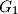$G_1$ and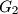$G_2$ intersect at two points$M$ and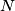$N$. Let$AB$ be the line tangent to these circles at$A$ and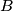$B$, respectively, so that$M$ lies closer to$AB$ than$N$. Let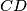$CD$ be the line parallel to$AB$ and passing through the point$M$, with$C$ on$G_1$ and$D$ on$G_2$. Lines$AC$ and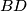$BD$ meet at$E$; lines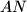$AN$ and$CD$ meet at$P$; lines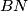$BN$ and$CD$ meet at$Q$. Show that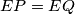$EP = EQ$.
Izvor: Međunarodna matematička olimpijada, shortlist 2000